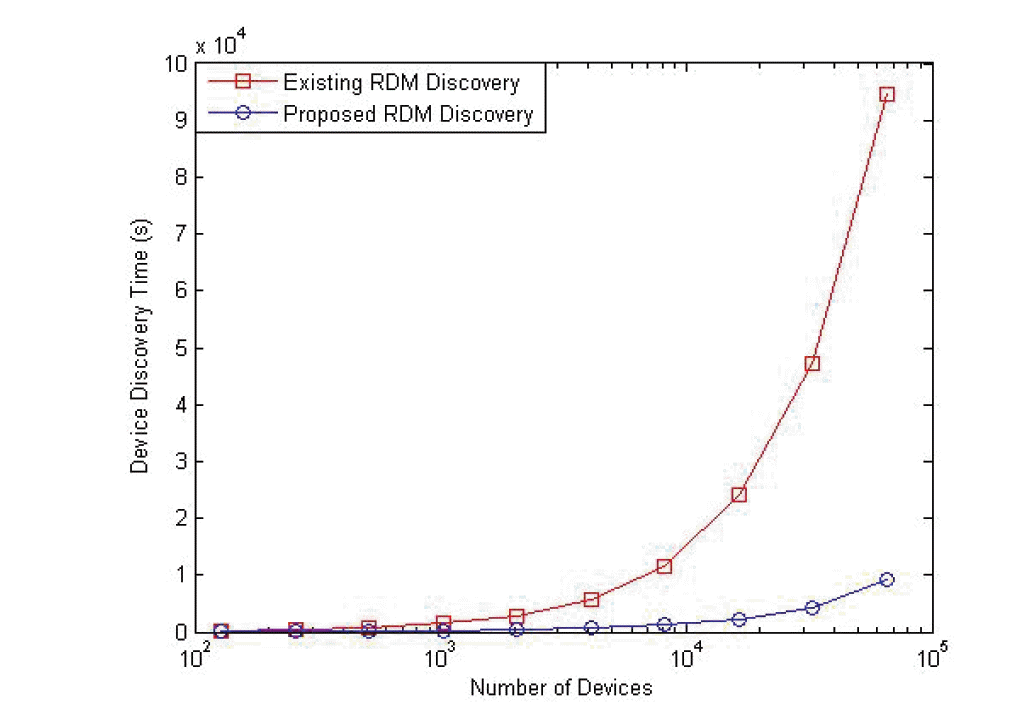Choi, Lee, Koh, Lim, Kim, and Kang: Fast Device Discovery for Remote Device Management in Lighting Control Networks

### Abstract

The Remote Device Management (RDM) protocol is used to manage the devices in the lighting control networks. RDM provides bi-directional communications between a controller and many lighting devices over the DMX512-A network. In RDM, using a simple binary search scheme, which is based on the 48-bit unique ID (UID) of each device, discovers the lighting devices. However, the existing binary search scheme tends to require a large delay in the device discovery process. In this paper, we propose a novel partition-based discovery scheme for fast device discovery in RDM. In the proposed scheme, all devices are divided into several partitions as per the device UID, and the controller performs device discovery for each partition by configuring a response timer that each device will use. From numerical simulations, we can see that there is an optimal number of partitions to minimize the device discovery time for a given number of devices in the proposed scheme, and also that the proposed partition-based scheme can reduce the device discovery time, as compared to the existing binary search scheme.

### 1. Introduction

One of the primary issues in the lighting control network is how to effectively manage a lot of devices within the network. Some protocols for device management have so far been made in the PLASA Technical Standards Program (TSP) , which includes the Digital Multiplex 512-A (DMX512-A)  and Remote Device Management (RDM) .
The RDM protocol is performed based on the polling system, in which a controller initiates communication and the devices will respond to the request of controller. RDM is used for the discovery and management of devices that are connected through the DMX512-A link. For device discovery, the controller uses a simple binary search scheme, based on the 48-bit unique ID (UID). However, this device discovery scheme tends to require a lengthy discovery time due to exploring the 248 theoretically possible devices, even though a controller is usually able to handle only tens of thousands.
In this paper, we propose a partition-based discovery scheme for fast device discovery in RDM. In the proposed scheme, all devices in the RDM network are divided into several partitions as per the device UID, and the controller performs the device discovery for each partition by using a suitable response timer for the devices.
This paper is organized as follows: Section 2 discusses the existing device discovery scheme. In Section 3, we propose the fast device discovery scheme for RDM. In Section 4, we analyze and compare the existing and proposed schemes in terms of device discovery times. Section 5 concludes this paper.

### 2. Existing Device Discovery Scheme

In RDM , a simple binary search scheme is used for device discovery, which is based on the 48-bit UID of each device. Fig. 1 shows the existing device discovery scheme, in which a controller performs a binary search for devices with the UIDs ranging from 0 to 248.
Initially, the controller sets the UID search range with (lower, upper) and sends a discovery message to all of the devices in the RDM network. If the controller responds to a single response message, the corresponding device will be successfully found. Otherwise, if two or more devices have responded at the same time, the controller cannot process the multiple response messages due to the characteristics of the DMX512-A link . This is because the DMX512-A link does not use the packet scheduling functionality and thus, multiple response messages will be simply corrupted and discarded by the controller. In this case, the controller will further divide the UID search range into the two parts: (lower, mid) and (mid+1, upper) as per the binary search algorithm, as shown in Fig. 1. This search process will be repeated, until all devices in the network are found.

### 3. Proposed Device Discovery Scheme

The existing device discovery scheme gives a lengthy device discovery time because the 48-bit UID search range (248) tends to induce very deep tree levels along the binary search tree. Thus, we propose a new device discovery scheme, called partition-based device discovery.
In the proposed scheme, all devices in the network will be grouped into Np partitions, as shown in Fig. 2.
Given the number of partitions (Np), the partition of a device (0, 1, .., Np-1) is determined by using the modulo (%) operator and the device UID, as follows:
PartitionofdeviceUID=UID%Np.
Then, the proposed scheme is performed for each partition, as shown in Fig. 3.
For each partition k, the controller first sends a discovery message to all devices. Then, all of the devices that belong to the partition shall respond to the controller. If two or more response messages are generated at the same time, the controller will fail to discover those devices due to collisions. Thus, to reduce the possibility of multiple responses, we employed the Maximum Response Timer (MRT) for devices. That is, when a device receives a discovery message from the controller, it responds to the controller only after a certain waiting time, which is randomly generated with a range of (0, MRT) by the device. This timer will facilitate in reducing the probability of multiple responses from many devices, and thus will increase the possibility of a single (successful) response.
In Fig. 3, the information on the current partition (Np and k) and MRT shall be included in the discovery message that is sent by the controller. The responses from devices can be classified into the following three cases:
• Fail Response, in which all of the responses have arrived at the controller at the same time and thus they are corrupted. The controller shall re-discover the devices after increasing the MRT timer by the Delay Increase Interval (DII).

• Success Response, in which some of the responses have arrived at the controller at different time intervals. However, the remaining devices shall be re-discovered.

• No Response, which means that there are no more devices to be discovered in the partition. Go to the next partition.

In the case of Fail Response, the controller adjusts the MRT timer to MRT + Delay Increase Interval (DII), and then sends a discovery message to the devices again, as shown in Fig. 3. In the Success Response case, some devices are found, but there are more devices to be discovered in the partition.
These procedures will be repeated for each partition, until all of the devices in the network have been discovered.

### 4. Performance Analysis

To evaluate the performance of the proposed scheme, we compared the device discovery time for the existing binary search scheme and the proposed partition-based scheme in a variety of network conditions. For numerical analysis, we used MATLAB (MathWorks, Natick, MA, USA), and we performed five test instances and obtained the averaged values in simulations.
For analysis, we set a unit transaction time for the discovery-response messages between the controller and devices as 1 second. In the proposed scheme, the unit for the time slot associated with the Maximum Response Time (MRT) and Delay Increase Interval was set as 0.0028 second by referring to . For example, a device responds to the controller after the MRT x 0.0028 seconds, when it receives a discovery message from the controller. The initial MRT is set to 10 time slots.
In our analysis, we first examined an optimal configuration of the parameters that are used in the proposed scheme, such as the Delay Increase Interval (DII) and the number of partitions (Np). After that, we compared the proposed scheme with the existing scheme in terms of device discovery time.
Fig. 4 shows the impacts of both Np and the number of devices on the device discovery time, in which DII is set to 10 time slots. It is noted in the figure that the device discovery time is plotted in the time unit of seconds, whereas, the basic operational time unit is 0.0028 seconds in each simulation. From this figure, we can see that the device discovery time tends go higher, as the number of devices increases. From the results, it is noted that there may be an optimal number of Np for a given number of devices. For example, for 210 devices, Np = 3 gives the best performance, whereas, the device discovery time can be minimized at Np = 6 when there are 212 devices in the network.
Fig. 5 shows the impacts of Np and DII on the device discovery time, in which the number of devices is given by 210. We see that Np = 3 is a reasonable choice for the best performance for all DIIs. On the other hand, it is shown that the device discovery time lessens, as the DII increases. This implies that a large DII (e. g., 200 or 500) can reduce the possibility of multiple responses (collisions) and thus increase the chances of success responses, as compared to a small DII (e.g., 50). However, as Np gets larger, the performance gaps for different DIIs are not significant, which implies that we need to conduct more experiments to obtain a more suitable configuration of the parameters.
Fig. 6 analyzes the relationship between DII and the number of devices, in which Np is given by 10. From the figure we can see that there is an optimal DII for a given number of devices. That is, DII = 200 is preferred for a small number of devices (4,000 or less), whereas, DII = 500 seems to provide better performance for a large number of devices (5,000 or more).
Fig. 7 analyzes Np for the different number of devices, in which DII is given by 10. In this figure, we can see that for a smaller number of devices (4,000 or less), Np = 3 is a reasonable choice, but Np = 10 gives the best performance for a large number of devices (5,000 or more). It is noted that the performance gaps for different Np becomes larger, as the number of devices increase.
Up until this point, we have investigated the optimal configuration of the parameters used in the proposed scheme through simulations. From the simulation results, we can see that the following relationship between the device discovery time and the protocol parameters exists: “device discovery time ∝ (number of devices * Np /DII).” That is, the device discovery time tends to be proportional to the number of devices and the number of partitions (Np), but inversely proportional to the DII.
With this obtained optimal configuration, we compared the overall device discovery time for the existing binary search scheme and the proposed partition-based scheme, as shown in Fig. 8. From this figure, we can see that the proposed scheme gives a much smaller device discovery time than the existing scheme does. Moreover, the performance gaps between the existing and proposed schemes become larger, as the number of devices increases. This is because the proposed scheme can reduce the attempts made for device discovery by dividing all devices into several partitions and can also minimize the possibility of multiple responses (collisions) from the devices, as compared to the existing binary search scheme.

### 5. Conclusions

In this paper, we have proposed a new partition-based device discovery scheme in lighting control networks. In the proposed scheme, all devices are divided into several partitions. In addition, to avoid collisions occurring due to multiple responses, each device sends a response message based on a response timer that is configured by the controller.
From the numerical analysis, we can see that the proposed scheme can provide a much lower device discovery time than the existing scheme. Moreover, the performance gaps between the existing and proposed schemes become lager, as the number of devices increase

### Acknowledgement

This research was supported by the ICT Standardization program of MSIP (Ministry of Science, ICT & Future Planning), and the Basic Science Research Program of NRF (2010-0020926).

### Biography

He received B.S. and M.S. degrees in School of Computer Science and Engineering from Kyungpook National University in 2010 and 2012, respectively. Since March 2012, he is with the School of Computer Science and Engineering from Kyungpook National University as a Ph.D. candidate.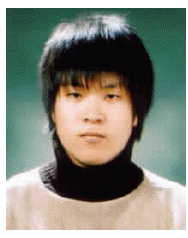### Biography

He received M.S. degree in School of Computer Science and Engineering from Kyungpook National University in 2014. His current research interests include mobile communication and lighting control network.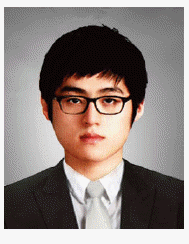### Biography

He received the B.S. and M.S. degrees in Management Science from KAIST in 1992 and 1994, respectively. He also received Ph.D. degree in Industrial Engineering from KAIST in 1998. From August 1998 to February 2004, he worked for Protocol Engineering Center in ETRI. He has been as a professor with the school of Computer Science and Engineering in the Kyungpook National University since March 2004. His current research interests include mobility management in IP mobility, multicasting, and SCTP.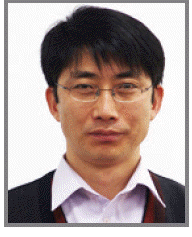### Biography

Sang-Kyu Lim
He is a principal member of engineering staff in the Electronics and Tele-communications Research Institute(ETRI) in Daejeon, Korea. He received his B.S. degree in Physics in 1995, and M.S. and Ph.D. degrees in Electronics Engineering in 1997 and 2001 from Sogang University, Seoul, Korea. Since he joined ETRI in 2001, he has worked on high-speed optical transmission systems and the microwave/millimeter-wave circuit design. He is concentrating in the areas of visible light communication and lighting control networks. He is one of the major contributors in developing the IEEE 802.15.7 standard and ANSI E1.45 standard on VLC as well as a senior member of the IEEE and a principal member of the PLASA CPWG.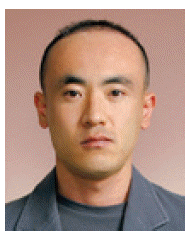### Biography

Insu Kim
He is a senior member of engineering staff in the Electronics and Telecommunications Research Institute(ETRI) in Daejeon, Korea. He received his B.S. degree in 2000 and M.S. and Ph.D. degrees in 2002 and 2008 in Computer Engineering from Konkuk University, Seoul, Korea. Since he joined ETRI in 2009, he has worked on LED lighting control systems and lighting control networks.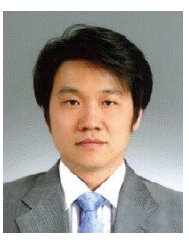### Biography

Tae-Gyu Kang
He is working as a director at the LED Communication Research Section in the Electronics and Telecommunications Research Institute(ETRI) in Daejeon, Korea. He received his B.S. and Ph.D. degrees in computer science in 1987 and 2002 from Kyonggi University and M.S. degree in computer science from Chung-Ang University. Since he joined ETRI in 1989, he has worked on CCS No.7 intelligent system and VoIP technologies. His current research interests are LED system lighting and visible light communication. He is one of the major contributors in developing the TTA standard and IEEE 802.15.7 standard on VLC.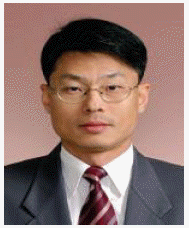### References

1. Professional Lighting And Sound Association (PLASA), http://www.plasa.org.

##### Fig. 1
Existing device discovery in Remote Device Management (RDM).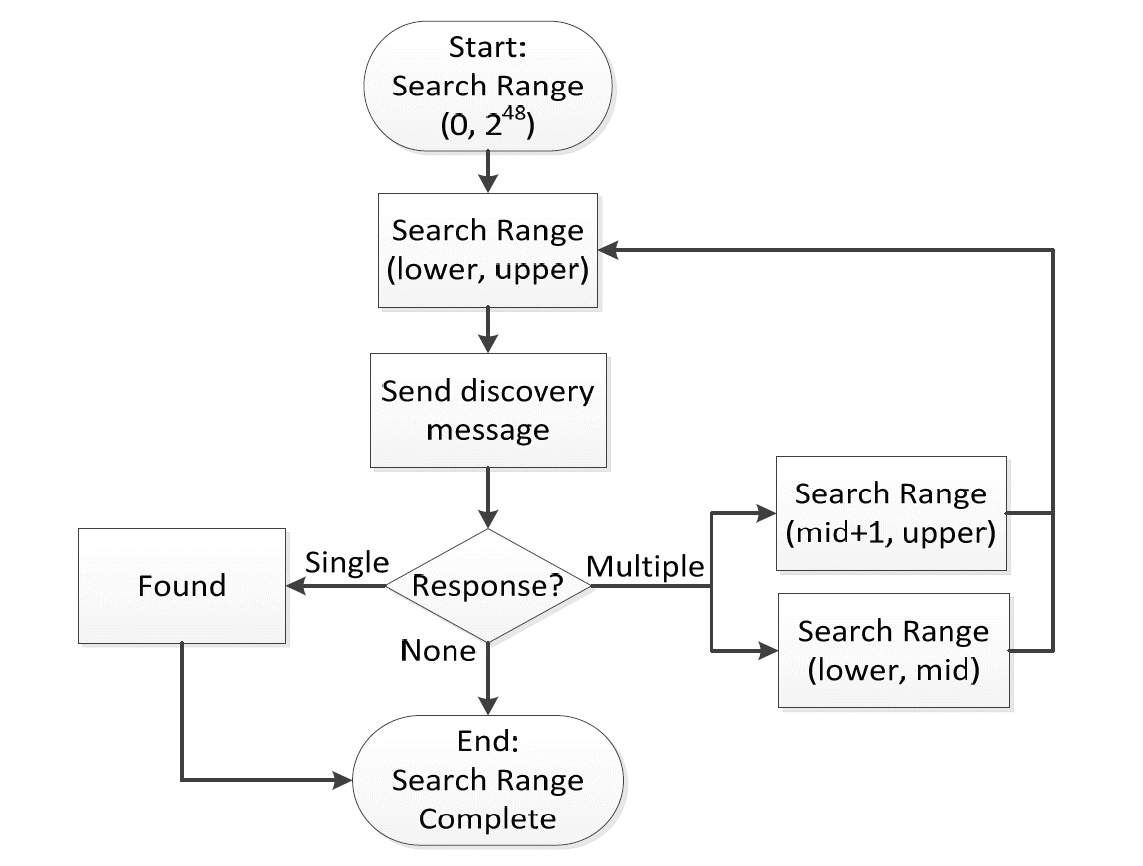##### Fig. 2
Partitions of devices in Remote Device Management (RDM).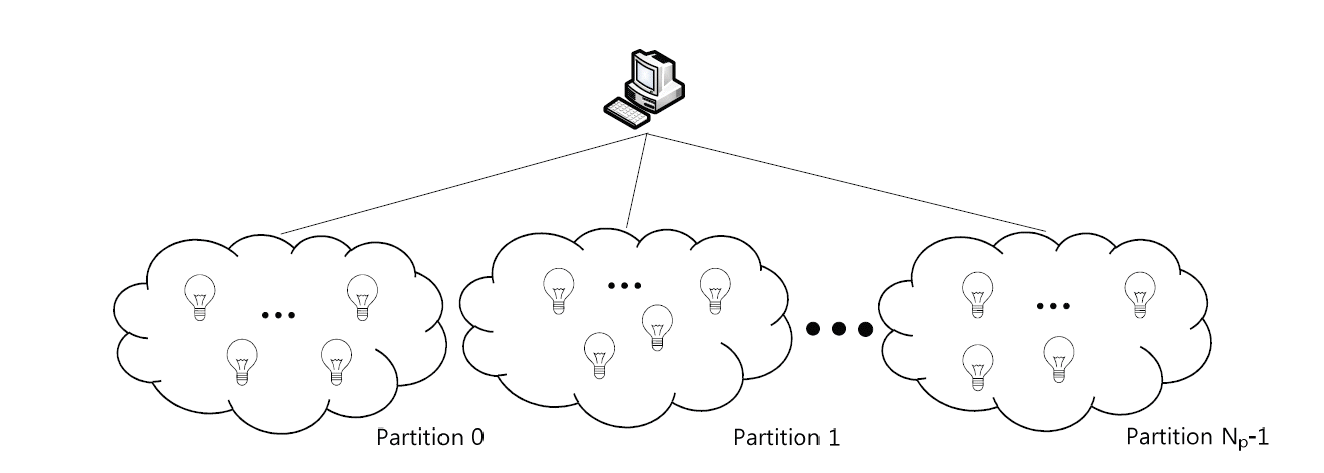##### Fig. 3
Proposed partition-based device discovery.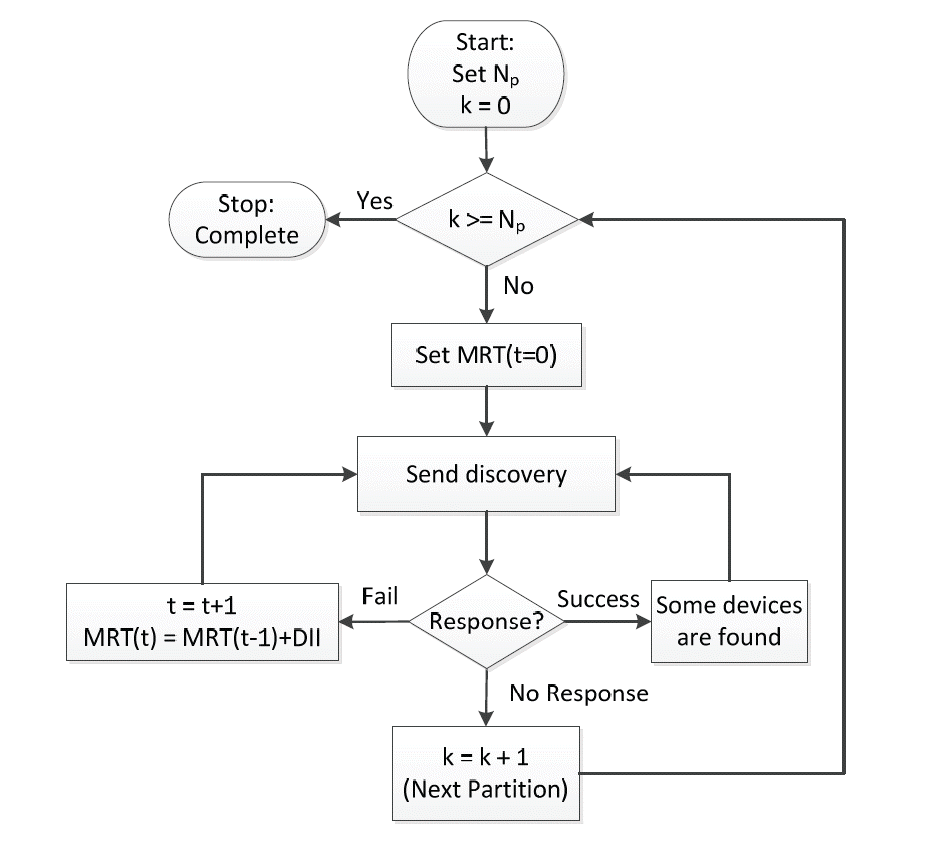##### Fig. 4
The impacts of both Np and the number of devices on the discovery time.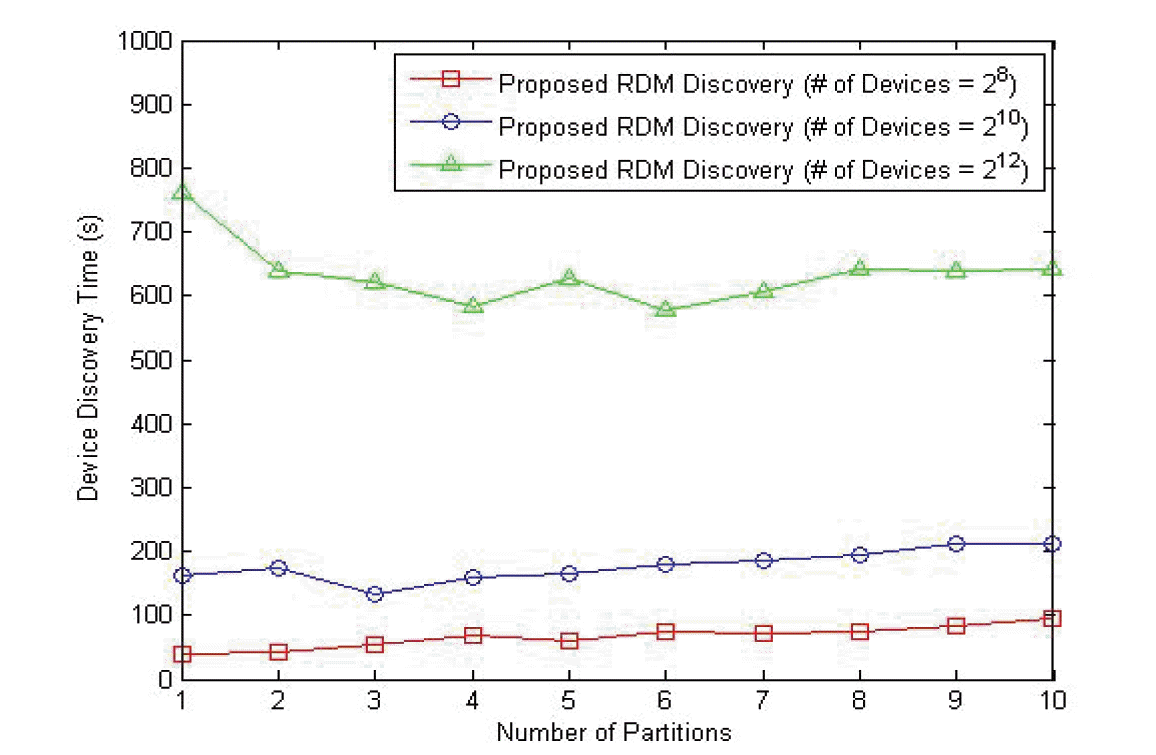##### Fig. 5
The impact of Np and DII on the proposed scheme.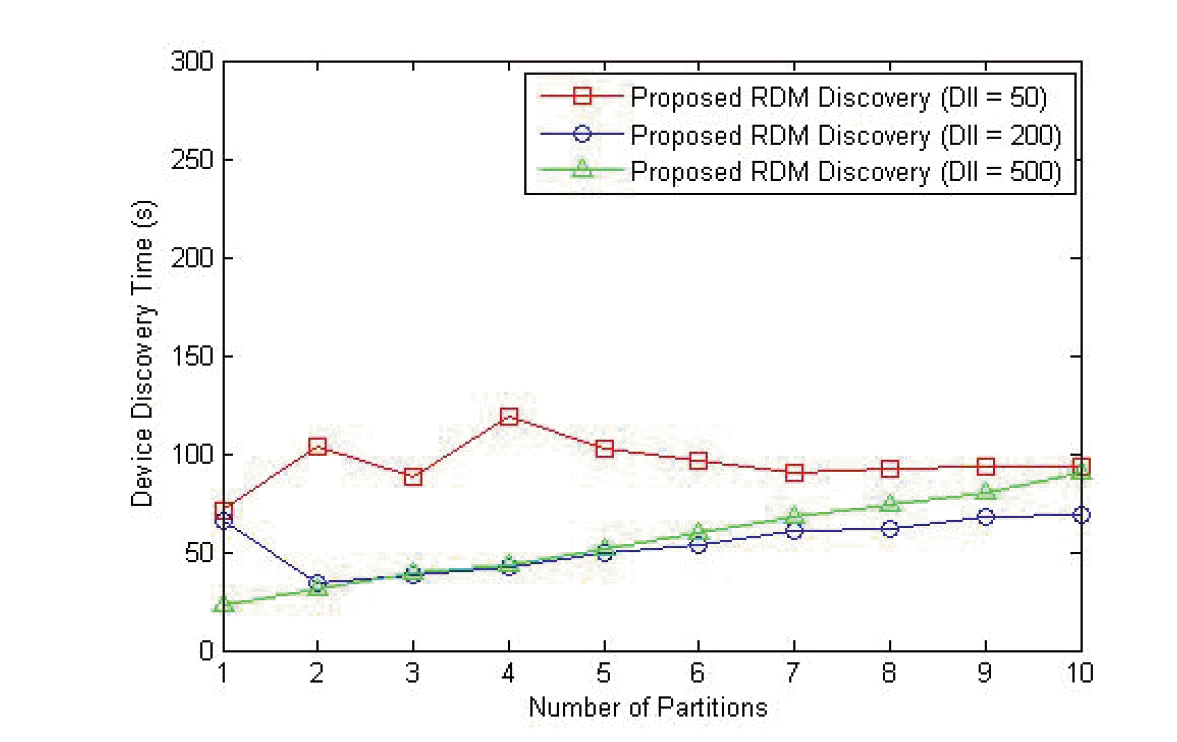##### Fig. 6
Analysis of DII by the number of devices.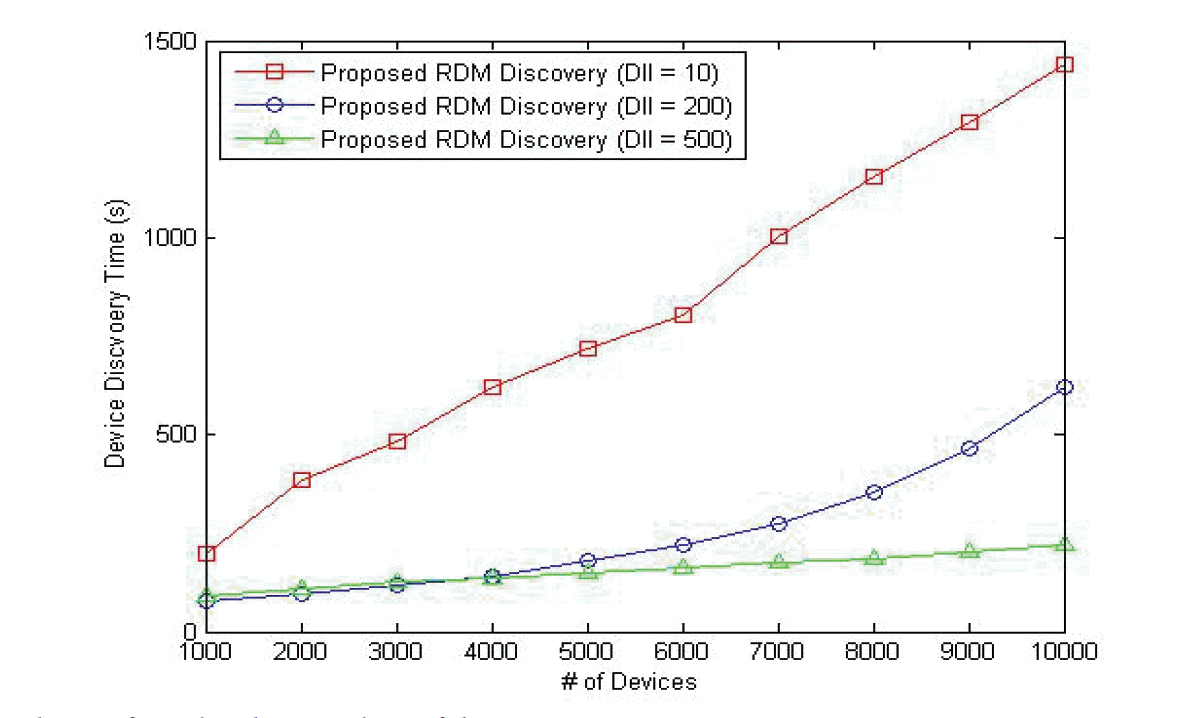##### Fig. 7
Analysis of NP by the number of devices.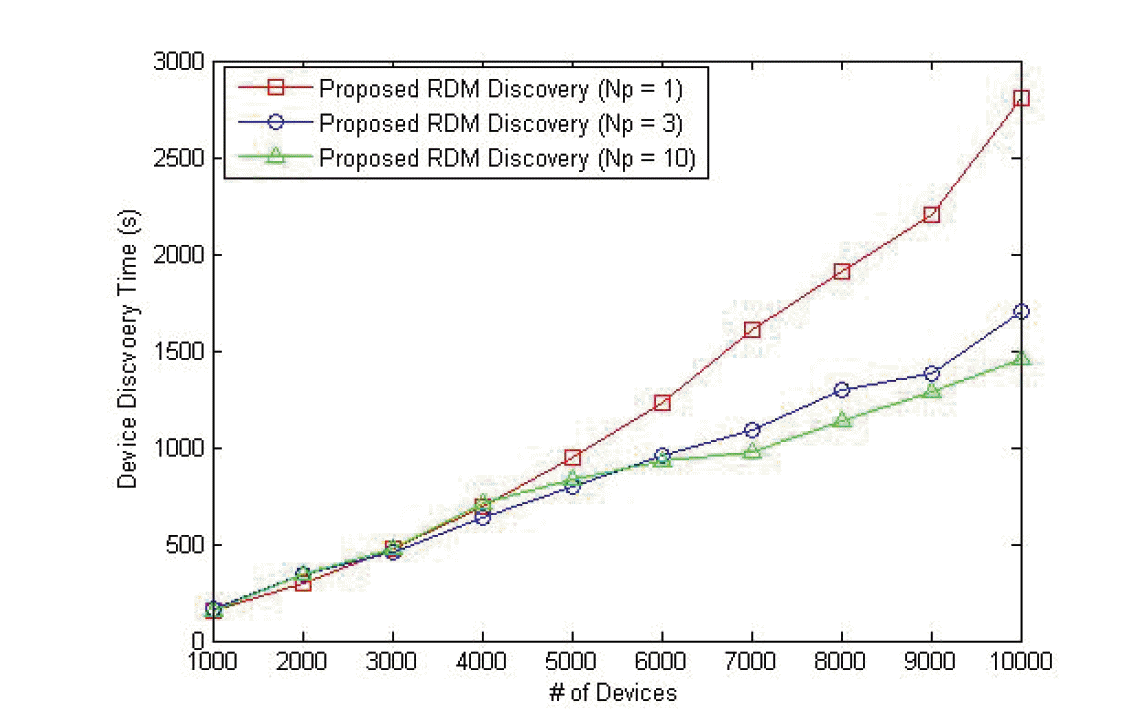##### Fig. 8
Comparison of existing and proposed schemes.